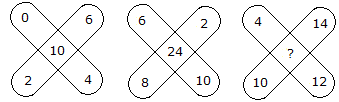# Verbal Reasoning - Character Puzzles - Discussion

### Discussion :: Character Puzzles - Character Puzzles 1 (Q.No.9)

9.

Which one will replace the question mark ?[A]. 36 [B]. 48 [C]. 38 [D]. 30

Explanation:

(0 + 2 + 6 + 4) - 2 = 10

and (6 + 2 + 10 + 8) - 2 = 24

Therefore, (4 + 14 + 12 + 10) - 2 = 38.

 Hari said: (Aug 24, 2013) Why we do minus 2 what is logic behind this?

 Padmaja said: (Dec 23, 2013) ((0+4)-1)+((6+2)-1) = 10. ((6+10)-1)+((2+8)-1) = 24. ((4+12)-1)+((10+14)-1) = 38.

 Saifulla said: (Apr 14, 2015) Kindly provide me some complex question which may help me to improve my technical back ground. For ex puzzle, verbal etc.

 Vipin Singha said: (Apr 15, 2016) 24 - 10 = 14. 24 + 14 = 38.

 Mahesh Jadar said: (May 19, 2017) Superb, Thanks for the given explanation.

 Shivani Kukreja said: (May 25, 2017) I am not getting this, Will anyone please explain this question?

 Rupesh Sharma said: (May 27, 2017) 10 * 2 = 24 - 20 = 4. 24 * 2 = 48 - 10 = 38.

 Ajeet said: (Jun 25, 2017) (6+4)*1=10. (10+2)*2=24. (14+12)*3=38.

 Arjun Raghav said: (Jul 5, 2017) (0+2+6+4)-2=10. And (6+2+10+8)-2=24. There for (4+14+12+10)-2=38.

 Ajith C R said: (Jul 5, 2017) 14 common number. 10+14= 24. So 24+14=38.

 Shiv Charan Dilwale said: (Jul 6, 2017) (0+2+4+6)-2=10, (2+8+6+10)-2=24, so (4+12+14+10)-2 = 38.

 Anupam Gupta said: (Jul 8, 2017) BASICALLY, WE ANALYSE THE QUESTION. SEE, 0 + 2 + 6 + 4) - 2 = 10. and (6 + 2 + 10 + 8) - 2 = 24. Therefore, (4 + 14 + 12 + 10) - 2 = 38. IN THIS QUESTION, WE CAN ARRIVE AT 10 AND 24 ONLY AFTER WE DEDUCT 2. SO PATTERN FOLLOWS AND WE DO EXACTLY SAME THING AGAIN, IT CAN BE ANY NUMBER NOT ONLY 2.

 Gayu said: (Jul 26, 2017) Thank you for the given explanation.

 Pydi Raj said: (Aug 11, 2017) Nice explaination.

 Ranjit Kumar said: (Sep 6, 2017) (0+2+4+6) -2=10, (2+8+6+10) -2=24, so (4+12+14+10) -2 = 38.

 Sanjana said: (Oct 6, 2017) Thank you for the explanation.

 Arshia Bhansali said: (Oct 6, 2017) Nice explanation, Thank you all.

 Sanyam said: (Oct 8, 2017) Nice explanation. Thank you all.

 Pramod said: (Oct 22, 2017) 0+2+4+6=12-2=10, 6+8+10+2=26-2=24, 4+10+12+14=40-2=38.

 Ashok Kumar Sethy said: (Oct 24, 2017) I can't understand it. Please explain it.

 Mukund said: (Oct 26, 2017) Add all number and subtract 2 from it then you will get middle term.

 Prragya Bakshi said: (Oct 31, 2017) It was good. But please don't tell the answer.

 Vadthyavath Balu said: (Nov 7, 2017) 0+2+4+6=12-2=10, 6+8+10+2=26-2=24, 4+10+12+14=40-2=38.

 Homey said: (Nov 7, 2017) The answers and explanations are cool. No need giving mine.

 Pankaj Chaturvedi said: (Nov 21, 2017) ((0+6)+(2+4)-2)=10 ((6+2)+(8+10)-2)=24 ((4+14)+(10+12)-2)=38.

 Deepak Kumar said: (Dec 28, 2017) The Answer will be 36 every time we see the middle no is exactly divided by one no. And the middle is equal to the addition of the rest of the number.

 Arun Kumar Gaur said: (Jan 10, 2018) 6+4+0+2)-2=10. 8+2+10+6)-2=24. 10+4+14+12)-2=38.

 Rahul Anand said: (Jan 17, 2018) (6+4+0+2)-2=10. (8+2+10+6)-2=24. (10+4+14+12)-2=38.